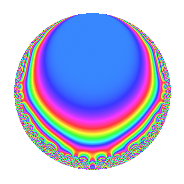Properties

 Label 17.4.bLevel 17 Weight 4 Character orbit b Rep. character $$\chi_{17}(16,\cdot)$$ Character field $$\Q$$ Dimension 4 Newforms 1 Sturm bound 6 Trace bound 0

Related objects

Defining parameters

 Level: $$N$$ = $$17$$ Weight: $$k$$ = $$4$$ Character orbit: $$[\chi]$$ = 17.b (of order $$2$$ and degree $$1$$) Character conductor: $$\operatorname{cond}(\chi)$$ = $$17$$ Character field: $$\Q$$ Newforms: $$1$$ Sturm bound: $$6$$ Trace bound: $$0$$

Dimensions

The following table gives the dimensions of various subspaces of $$M_{4}(17, [\chi])$$.

Total New Old
Modular forms 6 6 0
Cusp forms 4 4 0
Eisenstein series 2 2 0

Trace form

 $$4q$$ $$\mathstrut -\mathstrut 2q^{2}$$ $$\mathstrut +\mathstrut 2q^{4}$$ $$\mathstrut -\mathstrut 18q^{8}$$ $$\mathstrut -\mathstrut 40q^{9}$$ $$\mathstrut +\mathstrut O(q^{10})$$ $$4q$$ $$\mathstrut -\mathstrut 2q^{2}$$ $$\mathstrut +\mathstrut 2q^{4}$$ $$\mathstrut -\mathstrut 18q^{8}$$ $$\mathstrut -\mathstrut 40q^{9}$$ $$\mathstrut +\mathstrut 140q^{13}$$ $$\mathstrut +\mathstrut 24q^{15}$$ $$\mathstrut -\mathstrut 238q^{16}$$ $$\mathstrut +\mathstrut 112q^{17}$$ $$\mathstrut +\mathstrut 218q^{18}$$ $$\mathstrut -\mathstrut 112q^{19}$$ $$\mathstrut +\mathstrut 124q^{21}$$ $$\mathstrut -\mathstrut 460q^{25}$$ $$\mathstrut -\mathstrut 532q^{26}$$ $$\mathstrut +\mathstrut 912q^{30}$$ $$\mathstrut +\mathstrut 494q^{32}$$ $$\mathstrut -\mathstrut 1036q^{33}$$ $$\mathstrut +\mathstrut 406q^{34}$$ $$\mathstrut +\mathstrut 936q^{35}$$ $$\mathstrut -\mathstrut 218q^{36}$$ $$\mathstrut +\mathstrut 56q^{38}$$ $$\mathstrut -\mathstrut 1184q^{42}$$ $$\mathstrut -\mathstrut 520q^{43}$$ $$\mathstrut +\mathstrut 952q^{47}$$ $$\mathstrut +\mathstrut 312q^{49}$$ $$\mathstrut -\mathstrut 1354q^{50}$$ $$\mathstrut +\mathstrut 1160q^{51}$$ $$\mathstrut +\mathstrut 532q^{52}$$ $$\mathstrut -\mathstrut 576q^{53}$$ $$\mathstrut +\mathstrut 168q^{55}$$ $$\mathstrut -\mathstrut 1176q^{59}$$ $$\mathstrut -\mathstrut 912q^{60}$$ $$\mathstrut +\mathstrut 1426q^{64}$$ $$\mathstrut +\mathstrut 1904q^{66}$$ $$\mathstrut -\mathstrut 240q^{67}$$ $$\mathstrut -\mathstrut 406q^{68}$$ $$\mathstrut +\mathstrut 1204q^{69}$$ $$\mathstrut +\mathstrut 192q^{70}$$ $$\mathstrut -\mathstrut 1206q^{72}$$ $$\mathstrut -\mathstrut 56q^{76}$$ $$\mathstrut +\mathstrut 868q^{77}$$ $$\mathstrut -\mathstrut 2408q^{81}$$ $$\mathstrut -\mathstrut 2856q^{83}$$ $$\mathstrut +\mathstrut 1184q^{84}$$ $$\mathstrut +\mathstrut 768q^{85}$$ $$\mathstrut -\mathstrut 2248q^{86}$$ $$\mathstrut -\mathstrut 168q^{87}$$ $$\mathstrut +\mathstrut 1988q^{89}$$ $$\mathstrut +\mathstrut 1060q^{93}$$ $$\mathstrut +\mathstrut 448q^{94}$$ $$\mathstrut +\mathstrut 306q^{98}$$ $$\mathstrut +\mathstrut O(q^{100})$$

Decomposition of $$S_{4}^{\mathrm{new}}(17, [\chi])$$ into irreducible Hecke orbits

Label Dim. $$A$$ Field CM Traces $q$-expansion
$$a_2$$ $$a_3$$ $$a_5$$ $$a_7$$
17.4.b.a $$4$$ $$1.003$$ 4.0.4669632.2 None $$-2$$ $$0$$ $$0$$ $$0$$ $$q+(-1+\beta _{2})q^{2}+\beta _{1}q^{3}+(1-\beta _{2})q^{4}+\cdots$$